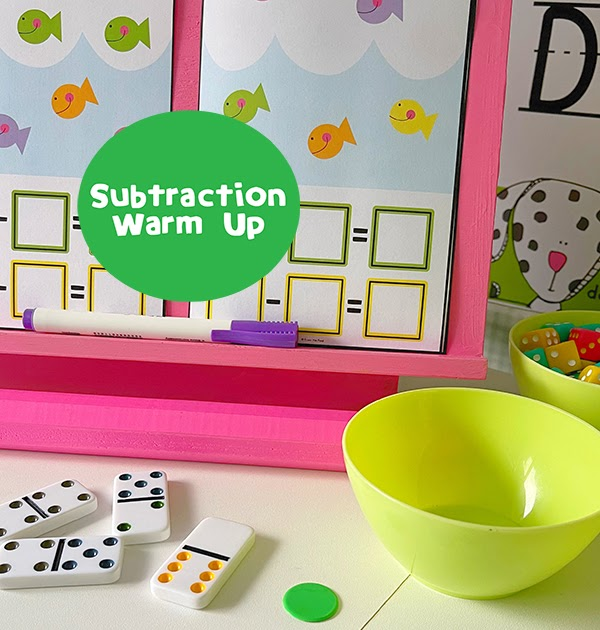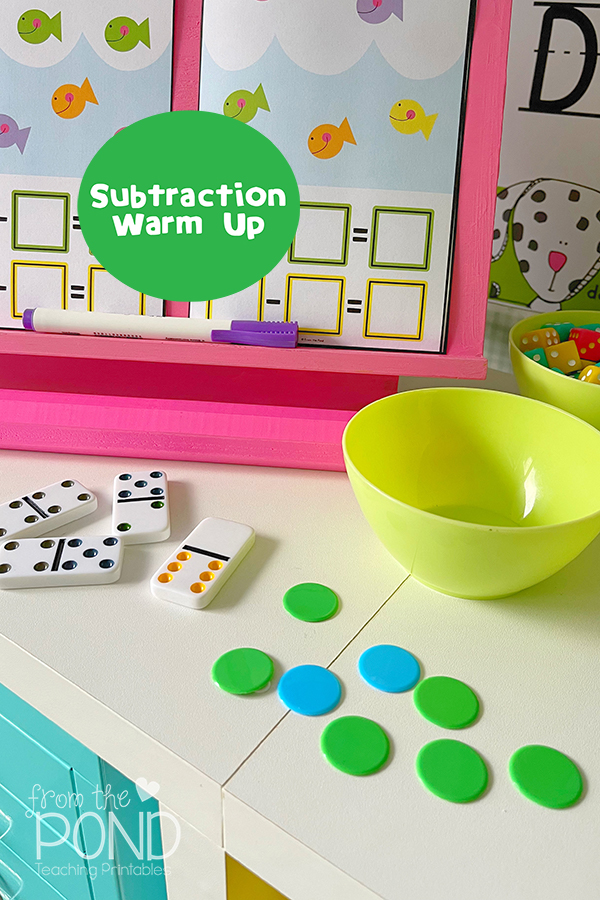Teaching

# Subtraction Fact Warm UpHere is a math warm up for subtraction. It can also be used as a lesson introduction or mini math workshop! Students will understand that from any 2 numbers, 2 subtraction stories (or facts) can be created. You just need:

• counters in 2 colors
• a dish
• dominosModel several first, talking about what you are doing and building an understanding of the key concepts.

Students then:

• take a domino
• tell the 2 numbers – here we have 5 and 2
• total the domino – 5 and 2 makes 7 altogether
• build the numbers with counters (this is important)

One of the games in our Subtraction Problem math pack will help students build a deeper understanding of related subtraction facts. It is called In and Out and each activity card shows fish in and out of the ocean!

Students can

• describe the subtraction story
• model it with counters on the card
• perform the subtraction (moving counters off the card)
• record it in numbers (directly on the card or on a whiteboard/paper)
Find the pack (which also includes activities to scaffold this concept) over in our store!

You may also like: